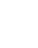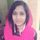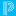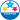DATsJourneyman

DATs

Hi,

is this the only way to get data from DATs. If one changes the timetable then one has to change the report tags. Not the right solution if someone can help.............

<tabl 2.7>^(*period_info;P2(I) P3(J) P4(E,G,H) P6(C) P7(B);current_percent)<tabl 3.75>^(*period_info;P2(F) P4(A,C) P6(E,H,I);current_percent)<tabl 4.54>^(*period_info;P2(B) P5(D) P6(A) P7(E,F) P8(I);current_percent)<tabl 5.30>^(*period_info;P2(A,E,J) P3(C) P4(F) P5(H) P6(G) P7(I) P8(B);current_percent)<tabl 6.30>^(*period_info;P3(G) P5(B,E) P7(J);current_percent)<tabl 7.30>^(*period_info;P2(H) P3(B) P5(F,J) P8(A);current_percent)<tabl 8.30>^(*period_info;P2(H) P3(B) P5(F,J) P8(A);current_percent)<tabl 9.30>^(*period_info;P2(D) P4(I) P7(A) P8(E,G);current_percent)<tabl 10.30>^(*period_info;P2(G) P3(F) P5(A,C) P6(B) P8(J);current_percent)<tabl 11.30>^(*period_info;P3(A,E,H) P4(J) P6(F) P8(D);current_percent)

Thank you.

5 RepliesCommunity Support Expert

Hi @pankaj

We recommend looking at your transcript objects which would help you in getting the data you are looking for. If you are looking for something else would you please provide us with more information such as what information are you trying to pull?

Saffiya Tasneem
Community Moderator

If a suggestion helps solve your issue, please select Accept As Solution on the post so others can see the solution, too!Journeyman

marks from examinationsPowerSchool Champion

@pankaj  The Transcript Object in an Object Report can pull whatever store code you'd like. If, like many Districts in New York, you use a store code of R6 for a June Regents Exam (State Test), you can have the Transcript Object pull an R6 store code.

______________
Full Disclosure: I do not work for PowerSchoolJourneyman

I am attaching a picture to explain what I am trying? I have mentioned the formula I am using (average formula). Is it the correct way of using it. I not then what is wrong?

<tabl 13.30>average(^(*period_info;P2(I) P3(J) P4(E,G,H) P6(C) P7(B);current_percent), ^(*period_info;P2(F) P4(A,C) P6(E,H,I);current_percent),^(*period_info;P2(B) P5(D) P6(A) P7(E,F) P8(I);current_percent), ^(*period_info;P2(A,E,J) P3(C) P4(F) P5(H) P6(G) P7(I) P8(B);current_percent), ^(*period_info;P3(G) P5(B,E) P7(J);current_percent), ^(*period_info;P2(H) P3(B) P5(F,J) P8(A);current_percent), ^(*period_info;P2(H) P3(B) P5(F,J) P8(A);current_percent), ^(*period_info;P2(D) P4(I) P7(A) P8(E,G);current_percent), ^(*period_info;P2(G) P3(F) P5(A,C) P6(B) P8(J);current_percent), ^(*period_info;P3(A,E,H) P4(J) P6(F) P8(D);current_percent))Community Support Expert

Hi @pankaj

We would need to take a closer look at your setup, so we recommend you reach out to your district's Designated Support Contact(DSC), who should be able to connect with the PowerSchool Support team in order to assist you further.

Saffiya Tasneem
Community Moderator